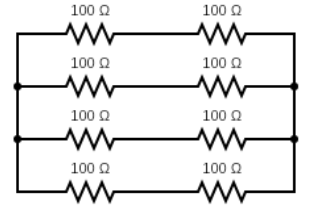Question

# A composite resistance of $50ohm$ which can carry a current of $4A$ is to be made from resistances each of resistance $100ohm$ which can carry a current of $1A$. the minimum number of resistances to be used is:

A

$4$

No worries! We‘ve got your back. Try BYJU‘S free classes today!
B

$8$

Right on! Give the BNAT exam to get a 100% scholarship for BYJUS courses
C

$12$

No worries! We‘ve got your back. Try BYJU‘S free classes today!
D

$16$

No worries! We‘ve got your back. Try BYJU‘S free classes today!
Open in App
Solution

## The correct option is B $8$Step 1: Given dataComposite resistance = $50\mathrm{\Omega }$Current through $50\mathrm{\Omega }=4\mathrm{A}$ Value of each resistance $=100\mathrm{\Omega }$Current through each $100\mathrm{\Omega }$ resistor$=1A$Step 2: Formula used$\frac{1}{{R}_{eq}}=\frac{1}{{R}_{1}}+\frac{1}{{R}_{2}}+\frac{1}{{R}_{3}}+\dots +\frac{1}{{R}_{N}}$ Where, ${R}_{eq}$ is the equivalent resistance and ${R}_{1},{R}_{2},{R}_{3},.....{R}_{N}$are the values of ${1}^{st},{2}^{nd},{3}^{rd},...{n}^{th}$resistance respectively.Step 3: Find the minimum number of resistances to be usedIf we consider $4$ pairs of resistances connected in parallel. The value of each pair will be $200\mathrm{\Omega }$.Then the equivalent resistance will be,$\frac{1}{{R}_{eq}}=\frac{1}{{R}_{1}}+\frac{1}{{R}_{2}}+\frac{1}{{R}_{3}}+\frac{1}{{R}_{4}}$Substituting the values in above equation we get, $\frac{1}{{R}_{eq}}=\frac{1}{200}+\frac{1}{200}++\frac{1}{200}++\frac{1}{200}$$⇒\frac{1}{{R}_{eq}}=\frac{4}{200}$ $⇒{R}_{eq}=\frac{200}{4}$$⇒{R}_{eq}=50\mathrm{\Omega }$Now, as the resistances are connected in parallel. The total current entering the system will be equal to the current leaving the system. Thus, the total current flowing through these $8$ resistors will be $4A$.Therefore, the minimum number of resistances to be used is $8$.Hence, option (B) is the correct answer.Suggest Corrections0Similar questionsExplore more